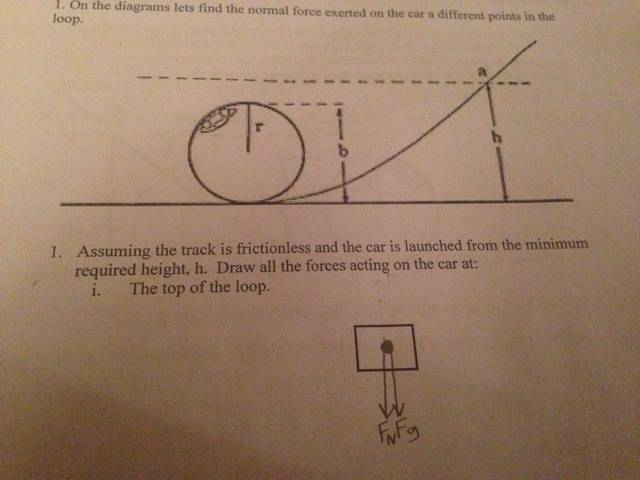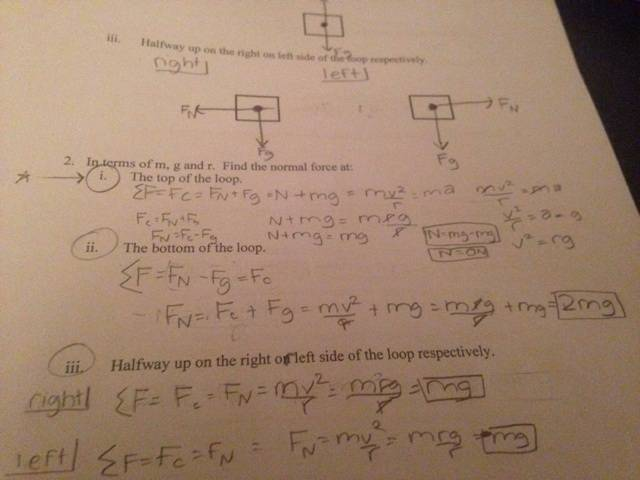# Rollercoasters and Loops and Normal Forces

## Homework Statement

<I will attach a diagram below> In terms of m, g and r. FInd the normal force at the top of the loop.

## Homework Equations

∑F = Fc = FN+Fg ∴ Fc=FN+Fg ∴FN = Fc - Fg ∴ N=mV2/r - mg

## The Attempt at a Solution

Fc=mV2/r - mg and V2=ra and a=g ∴C=mrg/r ∴C = mg ∴mg-mg=N ∴ N=0 N. BUT after discussing with a guy in AP Physics C at my school, he said that this is solving for the minimum height. SO I think I need to solve for the general equation to solve for the normal force at the top of the loop at any height using the variables m, g, and r only (I'm pretty sure that's what my instructions meant). Could anyone help please? I will send an image of my work as well.